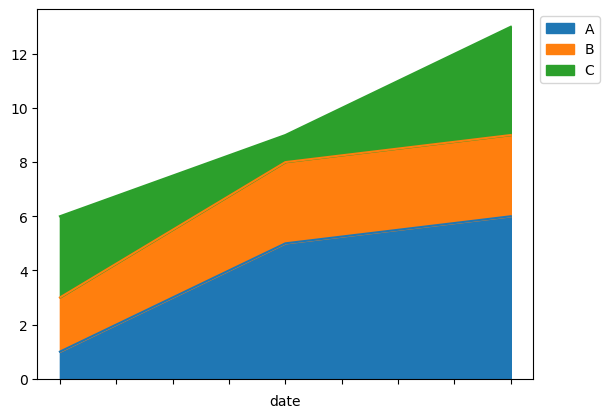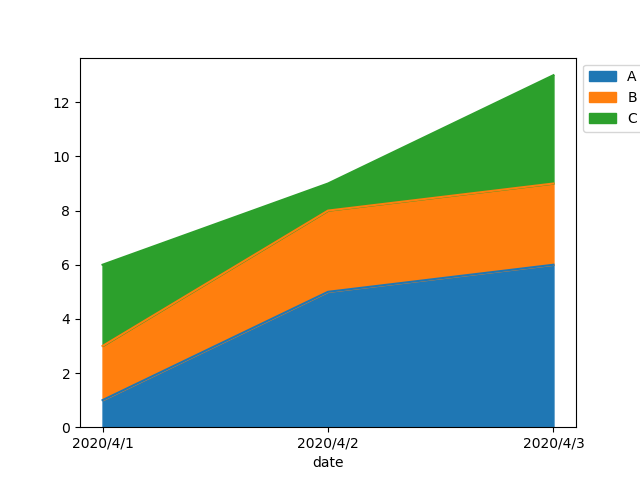•闪存
•博客
• 发言小组
• 投递新闻
• 提问博问
• 添加收藏
• 发布招聘
•文库

# 【python】请问画图如何设定x轴？

0悬赏园豆：30 [已解决问题] 解决于 2020-08-19 16:41``````name,date,A,B,C
one,2020/4/1,1,2,3
one,2020/4/2,5,3,1
one,2020/4/3,6,3,4
``````

x轴的地方是“date”，但我想要把x轴上的刻度用日期“2020/4/1”等显示出来。``````import pandas as pd
import matplotlib as mpl
import matplotlib.pyplot as plt
import datetime

fileName = 'test_01.csv'

df.set_index(df['date']).plot.area()
plt.legend(loc='upper left', bbox_to_anchor=(1, 1))
plt.savefig(fileName.replace('.csv','.png'), bbox_inches='tight')
``````

0

``````import pandas as pd
import matplotlib as mpl
import matplotlib.pyplot as plt
import datetime

fileName = 'test_01.csv'

df.set_index(df['date']).plot.area()
plt.legend(loc='upper left', bbox_to_anchor=(1, 1))
date_list = df['date'].tolist()
date_index = range(len(date_list))
plt.xticks(date_index, date_list, rotation=0)

plt.show()
``````@芽衣: 点我博客加微信,吧csv 和你写的代码发一下,我直接改一下给你

@小小咸鱼YwY: 你好，我在国外没有用微信欸。以前在国内申请过QQ，我用QQ加你吧。

@芽衣: 也可以,不过思路也可以告诉你,其实很简单再画图前你先自己对数组进行排序就可以了

@小小咸鱼YwY: 我已经在QQ上加你咯。排序的方法我之前有想过，但是最后弄出来的结果是

您需要登录以后才能回答，未注册用户请先注册